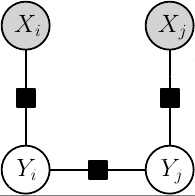grante
Inference and Estimation for Discrete Factor Graph ModelsConditional factor graph model

## Introduction

The grante library is a complete solution for probabilistic discrete factor graph models. In the library factor graphs specify probabilistic distributions over sets of discrete random variables. The distributions can be parametrized in a flexible way and these parameters can be estimated from training data. To make predictions using factor graphs the library contains a large number of different inference methods for both probabilistic inference of marginal distributions and for discrete energy minimization to infer the most likely configuration of unobserved variables.

For a detailed description of most of the algorithms implemented, please refer to the publications section.

## Features

grante includes source codes for the following functionalities:

### General

• Factor graph representation for discrete state models
• Unary, pairwise, and high-order factors
• Support for linear data-dependent factors and parameter sharing in factors
• Support for complex tying patterns within factor energies (symmetry, sparse energy tables, Potts, etc.)
• Support for non-linear data-dependent factors (e.g. RBF networks)
• Support for sparse factor data and for shared data among multiple factors
• Multi-core support through OpenMP
• Few dependencies: a C++0x compiler (MSVC 2010 or GCC 4.5) is needed to compile the library, and boost, version 1.40 or higher is required

### Inference

• Exact inference, sampling and MAP for tree-structured factor graphs
• Naive Gibbs sampling inference for general factor graphs
• Sum-product and max-product Loopy Belief Propagation, sequential and parallel schedules
• Structured Mean Field for v-acyclic decompositions
• Annealed Importance Sampling (AIS) inference for general factor graphs
• Generalized Swendsen-Wang MCMC inference for pairwise factor graphs where each variable has the same state space
• Approximate MAP-MRF Linear Programming inference (tree-based)
• Approximate MAP-MRF inference by simulated annealing
• Min-sum/sum-product diffusion
• Conditioning of distributions by discrete observations and factor-decomposable expectations

### Learning and Estimation

Parameters can be estimated for every model that can be specified in the library.

• Maximum (Conditional) Likelihood Learning for tree-structured factor graphs
• Maximum Pseudolikelihood Learning for general factor graphs
• Maximum Composite Likelihood Learning for general factor graphs specialized variant for 4-neighborhood grid graphs
• Contrastive divergence learning from fully observed and partially observed data
• Structured SVM for factor-decomposable loss functions (batch and stochastic training)
• Structured Perceptron (averaged)
• Prior distributions (and regularizers): multivariate Normal (L2), multivariate Laplace (L1), multivariate Student-t, multivariate Hyperbolic
• Expectation Maximization (EM) for partially observed data

### Optimization

• Barzilai-Borwein method for unconstrained differentiable minimization
• Limited memory BFGS method for unconstrained differentiable minimization
• FISTA composite minimization method for sparsity priors
• Approximately optimal v-acyclic decompositions of factor graphs
• Approximate tree-covering decomposition

### Interfaces

• Matlab interface for learning, inference, and sampling
• Native C++ interface

## Examples

Showcase of a few C++ API examples from the test cases.

```// ...
// Parameter estimation using Maximum Likelihood
Grante::MaximumLikelihood mle(&model);
mle.SetupTrainingData(training_data, inference_methods);
mle.Train(1e-5);
// ...

// ...
// Inference using loopy belief propagation
Grante::BeliefPropagation bpinf(&fg);
bpinf.PerformInference();
std::cout << "BP log_z " << bpinf.LogPartitionFunction() << std::endl;
const std::vector<double>& bp_fac0 = bpinf.Marginal(0);
// ...

// ...
// Empirical risk minimization training using Structured SVM
Grante::StructuredSVM ssvm_s(&model, 100.0, "bmrm");
ssvm_s.SetupTrainingData(instances, loss, inference_methods);
ssvm_s.Train(1e-4, 100);
// ...
```

Example use of the Matlab interface to sample from a 2D Ising model using Gibbs sampling.

```% Define model with a pairwise factor type
model=[];
model.factor_types(1).name = 'pw';
num_class=5;    % 5 labels for each pixel
model.factor_types(1).card = [num_class, num_class];

% Define pairwise Potts potential
strength = 1/3;
model.factor_types(1).weights = strength*ones(num_class,num_class);
model.factor_types(1).weights = model.factor_types(1).weights - ...
2.0*diag(strength*ones(num_class,1));

% Build factor graph
fg=[];
xdim = 64;
ydim = 64;
fg.card=num_class*ones(1, ydim*xdim);    % Set of random variables

% Instantiate pairwise factors
num_factors=(ydim-1)*xdim + (xdim-1)*ydim;
vfg=cell(1,num_factors);
fg.factors=struct('type',vfg,'vars',vfg,'data',vfg);
fi=1;
for yi=1:ydim
for xi=1:xdim
var_id=(xi-1)*ydim+yi;	% column-major
if yi > 1
% vertical pairwise edge
fg.factors(fi).type='pw';
fg.factors(fi).vars=[var_id-1, var_id];
fg.factors(fi).data=[];
fi=fi+1;
end
if xi > 1
% horizontal pairwise edge
fg.factors(fi).type='pw';
fg.factors(fi).vars=[var_id-ydim, var_id];
fg.factors(fi).data=[];
fi=fi+1;
end
end
end

% Produce 100 samples from the probability distribution
options=[];
options.gibbs_burnin = 1000;
options.gibbs_spacing = 100;
[states] = grante_sample(model, fg, 'gibbs', 100, options);
```

Distribution: source code, documentation, examples